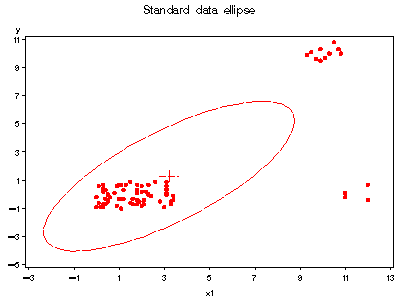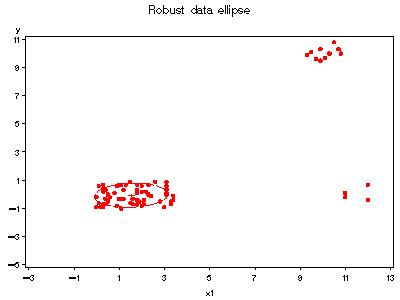robcov Calculate robust covariance matrix via MCD or MVE robcov

# SAS Macro Programs: robcov

\$Version: 1.3-2 (20 Mar 2007)
Michael Friendly
York University

## The robcov macro (get robcov.sas)

### Calculate robust covariance matrix via MCD or MVE

The ROBCOV macro calculates robust estimates of the mean vector, variance-covariance matrix and/or correlation matrix for a multivariate sample. It also calculates classical estimates of multivariate (Mahalanobis) distance, and robust estimates of multivariate distance which ignore potential outliers. Optionally, it produces a plot of robust vs. classical distances.

These results may be used both to detect multivariate outliers and leverage points, and to provide robust versions of any multivariate technique which depends on the covariance or correlation matrix, simply by substituting the robust result for the classical ones. Typical applications include regression, canonical correlation, factor analysis, and structural equation models, when the input may be supplied as a covariance (correlation) matrix, *or* when the procedure ignores observations with `WEIGHT=0 `to be excluded.

Two types of highly-robust estimators are provided: the minimum covariance determinant (MCD) estimator, based on the fast-MCD algorithm of Rousseeuw and Van Driessen (1999), and the minimum volume ellispoid (MVE) estimator based on earlier work by Rousseeuw (1984). MCD is defined as minimizing the determinant of the covariance matrix computed on h points, while MVE is defined as minimizing the volume of the p-dimensional ellipsoid containing h points.

### Method

The macro is essentially an easily-used interface to the SAS/IML MCD and MVE call routines. It creates a copy of the input variables augmented by the observation distances, robust distances, and weights. It creates robust covariance and correlation data sets in the form which may be used as input to other SAS procedures.

## Usage

The ROBCOV macro is defined with keyword parameters. There are no required parameters, but you should include an ID= variable for more interpretable results. The arguments may be listed within parentheses in any order, separated by commas. For example:

```  %robcov(data=hawkins, var=x1-x4 y, id=case);
```

### Parameters

DATA=
Name of the input data set [Default: `DATA=_LAST_`]
VAR=
List of input variable names. You may use any of the standard SAS abbreviations for variable lists, e.g., `VAR=X1-X20 Y1-Y3`, `VAR=AGE--STORAGE`, or `VAR=_NUMERIC_`. [Default: `VAR=_NUMERIC_`]
ID=
Name of an observation ID variable. This variable is simply copied to the output OUT= data set.
METHOD=
Robust estimation method: MVE or MCD [Default: `METHOD=MCD`]
OPT=
Options for the MVE or MCD call, a list of 5 numbers controlling the details MVE and MCD methods and printed output. See the SAS System Help (e.g., 'MCD call') for details on opt[]. The default, OPT=, is usually sufficient; two of these options may be specified more easily with the QUANTILE= and PRINT= options.
QUANTILE=
The quantile value of the h parameter, specified as a fraction of (n+p+1). This value may also be specified in terms of number of observations as the value of opt. If neither QUANTILE= or opt are specified, the default works out to `QUANTILE=.5` or h=(n+p+1)/2. This default has a high breakdown-bound (50%) but higher values, up to about `QUANTILE=.75` are more efficient under milder deviations from multivariate normality.
CORRECT=
Apply small sample and consistency corrections to the robust covariance matrix and robust distances? [Default: `CORRECT=Y`]
PRINT=
This parameter controls the printed output from the macro, and should be one of the following: NONE, SOME, ALL. Unless `PRINT=NONE`, opt is modified. [Default: `PRINT=SOME`]
OUT=
Output data set corresponding to the input DATA= data set, with classical Mahalanobis distances (DISTANCE), robust distances (RDIST), and observation weights (WEIGHT). [Default: `OUT=OUTDATA`]
OUTR=
Name for the output data set containing the robust correlation matrix, a `TYPE=CORR` data set. This data set is only produced if the OUTR= name is specified; if so, the macro fiddles with opt. [Default: OUTR=]
OUTC=
Name for the output data set containing the robust covariance matrix. [Default: `OUTC=OUTCOV`]
PLOT=
If non-blank, a plot RDIST * DISTANCE is produced, with reference lines and case labels identifying outliers. [Default: `PLOT=RDIST`]
SUBSET=
A logical expression to determine which observations are labeled in the plot. [Default: (`WEIGHT=0`)]
SYMBOL=
Value for point symbols in the plot. [Default: `SYMBOL=DOT`]

### Notes

The MCD estimator underestimates the scale of the covariance matrix, so the robust distances are slightly too large, and too many observations tend to be nominated as outliers. A scale-correction (Pison et al., 2002) has been implemented, and seems to work well enough to make `CORRECT=Y` the default.

For best results with the graphic plot, you should specify appropriate graphic options, such as `HTEXT=`, `FTEXT=`, `HTITLE=`, `FTITLE=` in a `GOPTIONS` statement.

## References

Pison, G., Van Aelst, S. & Willelms, G. (2002) "Small sample corrections for LTS and MCD," Metrika, in press.

Rousseeuw, P. J. (1984). ``Least median of squares regression,'' JASA, 79, 871-880.

Rousseeuw, P. J. & Van Driessen, K. (1990) ``A Fast Algorithm for the Minimum Covariance Determinant Estimator,'' Technometrics, 41, 212-223.

### Examples

This example uses the artificial data from Hawkins, Bradu, and Kass (1984), containing 75 observations on 4 variables. The first 10 observations are "bad leverage points," seriously affecting the regression of Y on X1-X3. The next 4 observations are "good leverage points," with high leverage on X1-X3, but small residuals on Y.
```%include macros(robcov);        *-- or include in an autocall library;

%include data(hawkins);
title 'Data from Hawkins et al.";
%robcov(data=hawkins, var=x1-x3 y, id=case, correct=N);
```
The default output contains the MCD results, the robust COV matrix, and the graph of robust vs. classical distance. See SAS Output from robcovt1.sas.

Note that two of the non-outliers are flagged with WEIGHT=0, as a result of the underestimate of scale. When re-run with the small sample corrections,

```%robcov(data=hawkins, var=x1-x3 y, id=case);
```
these cases are no longer flagged, and the robust distances are smaller. See SAS Output from robcovt2.sas.

Next, get the output data set from robcov and examine data ellipses

```%robcov(data=hawkins, var=x1-x3 y, id=case, out=hawkinsr);

axis1 order=(-5 to 11 by 2);
axis2 order=(-3 to 13 by 2);

title 'Standard data ellipse';
%ellipses(data=hawkinsr, y=y, x=x1, vaxis=axis1, haxis=axis2);

title 'Robust data ellipse';
%ellipses(data=hawkinsr, y=y, x=x1, weight=weight, vaxis=axis1, haxis=axis2);
```### See also

ellipses Plot bivariate data ellipses
outlier Robust multivariate outlier detection
robust M-estimation for robust models fitting via IRLS